Luo, L., Jin, Y., Li, M., Hu, L., Li, G., and Liu, Y. (2017). "Adsorption mechanism of anionic groups found in sulfonated mulberry stem chemi-mechanical pulp (SCMP) for removal of methylene blue dye," BioRes. 12(2), 2452-2465.

#### Abstract

Adsorption Mechanism of Anionic Groups Found in Sulfonated Mulberry Stem Chemi-Mechanical Pulp (SCMP) for Removal of Methylene Blue Dye

Lianxin Luo,a,b* Ye Jin,a,b Mingfu Li,a,b Lingyu Hu,a,b Guangxing Li,a,b and Yi Liu a,b

Keywords: Anionic groups; Methylene blue; Absorption thermodynamics; Kinetic model

Contact information: a: College of Light Industry and Food Engineering, Guangxi University, Nanning, 530004, P. R. China; b: Guangxi Key Laboratory of Clean Pulp & Papermaking and Pollution Control, Nanning 530004, P. R. China; *Corresponding author: lxluo919@hotmail.com

INTRODUCTION

Studies by Li et al. (2012) showed that alkaline hydrogen peroxide bleaching can form new anion groups and surface anion groups. It was shown that carboxylate groups in uronic acid are the main contributor for pulp fiber anionic groups. The oxidation of the lignin moieties results in some carboxylation of that fraction, which amounts to about 30% of the net charge for the oxidation process. The amount of AGs is greatly influenced by the chemi-mechanical pulping conditions. The dosage of alkali and sodium sulfite, as well as the pretreatment temperature, will affect the amount of lignin and hemicelluloses removed from chemi-mechanical pulps (CMPs) as dissolved degradation products (He 2015). The conditions also affect the amount of sulfonic acid groups contained in the fibers. It makes lignin sulfonate, it causes swelling and hydrolyzation, wood pieces become soft, and fiber dissociates easily for sulfonated chemi-mechanical pulp (SCMP). The yields and optical properties of SCMPs are similar to those of stone ground wood (SGW), thermomechanical pulp (TMP), and other mechanical pulps (Zhan 2009). The CMPs have fiber lengths and drainage properties that are closely related to the semi-bleached kraft and acid sulfite chemical pulps. The location and number of AGs in the SCMP fibers affect the pulp’s papermaking performance. In the process of sulfites, sulfonation contributes to softening of timber, which also affects the cohesion of the cell wall. It has a large impact on the paper strength and pulp refining. Konn et al. (2007) found that the formation of anionic groups will increase the energy consumption of refining. Surface anion groups affect the interactions between the pulp fibers and papermaking additives, as well as the fiber-to-fiber bonding strength in the sheet. Most research studies regarding AGs and pulp fibers have focused mainly on carboxylate groups, and how these groups affect pulping and papermaking. The systemic research of carboxylates, sulfonates, total anion groups, and surface anion groups are rarely reported. Various adsorption isotherm models have been used to describe the adsorption process (Zhang 2009). The main focus of this paper is to study the adsorption of methylene blue (MB) by the AGs found in the SCMPs of mulberry stems, using unbleached SCMP to study MB adsorption isotherm experiments, as well as using a peroxide bleached SCMP to study MB adsorption kinetics.

EXPERIMENTAL

Materials

Whole mulberry stems were pulped by the SCMP process and bleached using hydrogen peroxide (H2O2). The pulp was refined to 44 °SR (Schopper-Riegler) freeness; the fibers had an average length of 0.58 mm and width of 16.02 μm. The unbleached SCMP brightness was 39.4% ISO, and the brightness of bleached pulp was 71.3% ISO (International Standard Organization). An analytical grade of methylene blue (MB) (Sigma-Aldrich, Shanghai, China) was used in the adsorption experiments

Methods

An adsorption isotherm was obtained by measuring the filtrate concentration (Ce) and adsorption equilibrium (Qe) at different temperatures. Into a 100-mL bottle with a stopper was added 100 mg of pulp and 50 mL of MB solution (100 mol∙L-1, 150 mol∙L-1, 200 mol∙L-1, 300 mol∙L-1, 350 mol∙L-1, or 400 mol∙L-1) with a pH of 8.00. The mixture was allowed to sit for 30 min at a temperature of 20 °C, 30 °C, or 40 °C. The adsorption equilibrium basically reached within 30 min; afterwards, the mixture was filtered to obtain the filtrate. The filtrates were diluted to a prescribed level so that absorbance was within the range of the ultraviolet–visible spectroscopy (UV/Vis) standard curve. The UV/Vis absorption of the solution was measured at the 664 nm wavelength. The amount of MB in the filtrate was determined from UV/Vis absorption based on the dilution level and a calibration curve. Equation (1) was used to calculate the equilibrium concentration, Ce, of MB and the equilibrium adsorption, Qe, of the adsorbent,

Qe = (CoCe)∙V/M (1)

where Qe is the adsorption capacity of the anionic groups of SCMP for MB at equilibrium (µmol∙g-1), C0 is the initial concentration of MB solution (µmol∙g-1), Ce is the concentration of MB solution at equilibrium (µmol∙g-1), V is the volume of the MB solution (L), and M is the mass of the SCMP absorbent (g).

Bleached pulp (100 mg; treated with 10% H2O2) was added to 200 mL of MB solution (500 µmol·L-1) with constant stirring at 20 °C and a pH of 8.00. The supernatant liquor was sampled and its UV/Vis absorbance was measured at regular intervals to calculate the concentration, which was then used to determine the adsorbent adsorption kinetics.

RESULTS AND DISCUSSION

Adsorption Isotherm Characteristics of Mulberry Stem SCMP on MB

The adsorption of MB by mulberry stem SCMP (i.e., adsorbate) was non-linear (Fig. 1). Fardim and Holmbom (2003, 2005) reported that the MB adsorption by the pulp fibers was non-linear. The adsorption data using bleached SCMP were regressed to the Langmuir and Freundlich isothermic models. The results from these curve fittings were compared.Langmuir and Freundlich models

A basic assumption of the Langmuir model is that adsorption occurs at specific sorbent sites, and that all the adsorption sites are energetically equivalent. The model also assumes that adsorption occurs as an ideal state. The regression results to obtain the equation parameter values of the Langmuir model are shown in Fig. 2 and Table 1. The Langmuir isotherm equation is expressed as,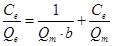(2)

where Qe is the adsorption capacity of the anionic group for MB at equilibrium (µmol∙g-1), Ce is the concentration of MB solution at equilibrium (µmol∙g-1), Qm is the the maximum adsorption capacity (μmol∙g-1), and b is the Langmuir constant.

The Freundlich isotherm model was established as an empirical approach in 1906 by Herbert Freundlich (Gao 2010). This empirical adsorption model can be used for non-ideal adsorption. Regression results to determine the parameter values of the Freundlich model for the adsorption of MB onto bleached SCMP is shown in Fig. 3 and Table 2. The Freundlich isotherm model can be expressed as,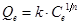(3)

and Eq. 3 can be written in logarithmic form as a linear equation,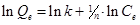(4)

where k is the adsorption equilibrium constant, and n is a model constant that is a function of temperature, adsorbent, and adsorbate species. The n parameter represents the sorption intensity of the adsorbent towards the adsorbate.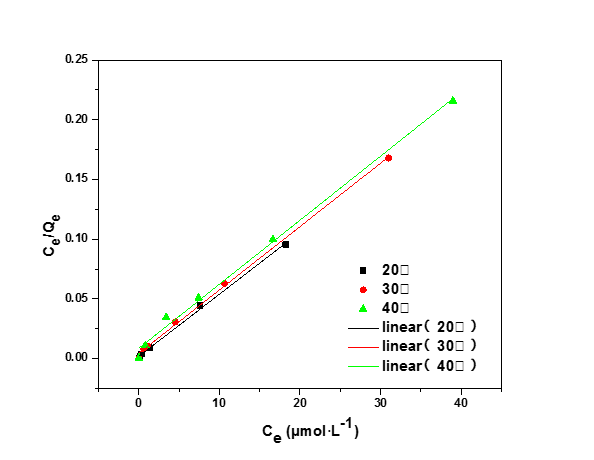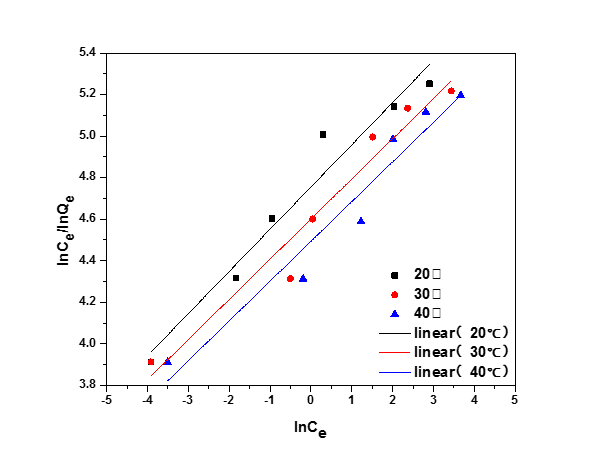The adsorption process could be described as having a uniform energy of adsorption (Langmuir) or as being heterogeneous (Freundlich). The Langmuir adsorption model (Fig. 2) fit the data to where the points fell linear. The Freundlich adsorption model (Fig. 3) showed that the data were relatively scattered around the linear regressions. From Tables 1 and 2, at a T of 293 K, 303 K, and 313 K, the coefficient of determination value (R2) of the Langmuir model was greater than 0.99, whereas it was between 0.93 and 0.96 for the Freundlich model. It was concluded that the Langmuir equation fit the data better than the Freundlich equation.

Table 1. Data Regression to Langmuir Isotherm Model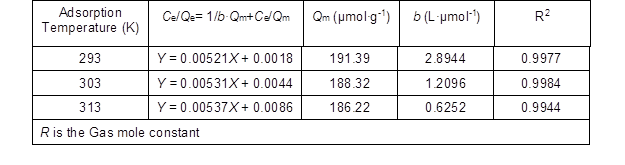Table 2. Data Regression to Freundlich Isotherm Model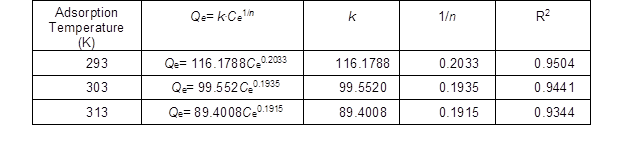The isotherm constant (b) of Langmuir adsorption reflects the relative affinity of adsorption sites for adsorbate molecules. Table 1 shows that the b values were lowered as the adsorption temperature increased, which indicated that the adsorption was reduced to a minor extent with higher temperatures. This indicates an increase in the adsorption energy of sites on the adsorbent surfaces on the MB molecules with decreasing temperature. Therefore, the maximum adsorption capacity (Qm) of the theoretical monolayer was increased with decreasing temperature.

The above analyses showed that the Langmuir model was a better fit to the isothermal adsorption data of MB onto SCMP than the Freundlich model. A dimensionless constant separation factor or equilibrium parameter KR can represent the basic characters of the Langmuir adsorption isotherm (Li et al. 2009; Hao et al. 2013; Kong et al. 2015). The KR parameter was defined as (Ho et al. 2002; Han et al. 2005),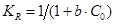(5)

where KR is the dimensionless separation factor, b is the Langmuir constant, and C0 is the initial MB concentration (µmol∙L-1).

The KR values determined the suitability of the Langmuir model to represent the adsorption data. When KR was greater than 1, the Langmuir model is not suitable. When KR is equal to 1, then the Langmuir model is linear. When the KR value is between 0 and 1, it indicates that the Langmuir model is suitable to represent the adsorption data. When KR is equal to 0, it indicates that the adsorption process is irreversible (Li et al. 2009). The KR values at different temperatures are shown in Table 3. In this part of the study, the KR values were between 0 and 1, which indicated that the Langmuir adsorption isotherm model was suitable for the MB adsorption process onto the SCMP of mulberry stems.

Table 3. Langmuir Isotherm KR Values at Different Temperatures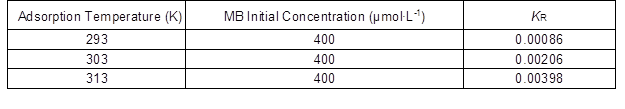The MB adsorption thermodynamics onto mulberry SCMP

When Gibbs free energy of the interaction between the adsorbate and the adsorbent is negative, it follows that adsorption takes place spontaneously. The process can be driven by a combination of enthalpy and entropy, including the effect of hydrophobic bond interactions that are driven by entropy. The adsorption process can be driven by a variety of forces, including mechanical action, electrostatic bonding, and chemical adsorption. Von Oepen et al. (1989, 1991) analyzed the various intermolecular forces that participated in the adsorption process: van der Waals forces, hydrophobic bonding, dipole-dipole interactions, hydrogen bonding, chemical bonding, and ligand exchange. Understanding these intermolecular forces involved in adsorption provided a theoretical basis for determining the thermodynamics that drive the process.

According to the calculation formula of Gibbs free energy, as well as the relationship with the thermodynamic equilibrium constant, the adsorption process can involve a change in free energy (Akhtar et al. 2006). The Gibbs free energy expression for adsorption is given as,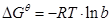(6)

The isobaric adsorption enthalpy can be written by the Van’t Hoff equation (Wei 2010),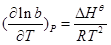(7)

The integration of this expression yields,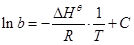(8)

The relationship between Gibbs free energy and enthalpy is given by,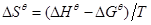(9)

where in these expressions, b is the Langmuir constant, R is the ideal gas law constant (8.314 J∙mol-1∙K-1), T is the absolute temperature (K) (i.e., 273+ temperature (°C)), and C is an integration constant.

Using Eqs. 6 and 9, one can calculate the Gibbs free energy (Gθ) and the reaction entropy (Sθ) for various temperatures. Plotting lnb versus 1/T (Fig. 4) and regressing the data to Eq. 8, one can determine the adsorption enthalpy (Hθ) from the linear slope of the regression equation. The adsorption thermodynamic parameters calculated from the linear regressions are shown in Table 4.Fig. 4. Relational graph between lnb and 1/T

Table 4. Thermodynamic Parameters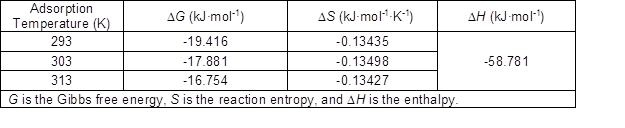The amount of Gibbs free energy, Gθ, for adsorption was less than zero (Table 4), indicating spontaneous adsorption. The Gibbs free energy of adsorption became less negative as the temperature was increased. This may be due to the presence of electrostatic attraction between the pulp fibers and MB, with increased temperature, the electrostatic attraction weakened.

The negative enthalpy value, H, indicated that the adsorption process was an exothermic process. The adsorption of MB onto the anionic groups of the pulp fibers resulted in the release of heat, whereas the desorption of MB (i.e., the reversed process) would be endothermic (i.e., adsorb heat). The calculated adsorption enthalpy was -58.781 kJ·mol-1, which was less than -30 kJ·mol-1; this showed that the MB adsorption process onto SCMP occurred concomitantly via physical and chemical processes.

MB adsorption kinetics onto mulberry stem SCMP

Adsorption kinetic models of ions can exhibit pseudo-first order or pseudo-second order mechanisms, or can be represented by an intra-particle diffusion model (Kumar et al. 2005; Lorenc-Grabowska and Gryglewicz 2005; Vadivelan and Kumar 2005; Zhang et al. 2012). The experimental data was further analyzed to determine which of these adsorption mechanisms of MB onto SCMP fibers best fit the process.

The pseudo-first-order adsorption kinetics model has been widely used to predict dye adsorption kinetics; the first-order equation is given as,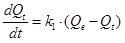(10)

This equation can be integrated and the logarithm taken to yield,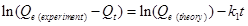(11)

The pseudo-second order adsorption kinetics model is expressed as,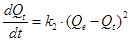(12)

Integration of Eq. 12 affords the following expression,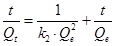(13)

At the initial adsorption time (t = 0), the initial adsorption rate, h (μmol∙g-1∙min-1), is represented as,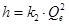(14)

The substitution of Eq. 14 into Eq. 13 yields the following expression,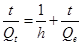(15)

The intra-particle diffusion model is given as,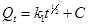(16)

where in these equations, Qt is the adsorption capacity (µmol∙g-1) of the SCMP for MB at time t, C is the integration constant, k1 is the rate constant of the pseudo-first order model (min-1), and k2 is the rate constant of the pseudo-second order model (g∙μmol-1∙min-1).

Linear regressions were performed on the data to Eqs. 11, 13, and 16, and the results are shown in Figs. 5, 6, and 7, respectively. The results from the regressions to determine the parameter values of the models are shown in Table 5.

Table 5. Kinetic Adsorption Equations and Parameters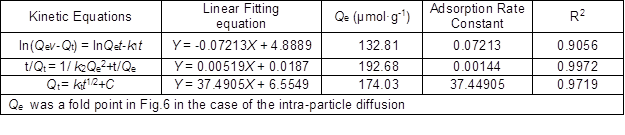Fig. 5. Data regression to pseudo-first-order modelFig. 6. Data regression to pseudo-second order modelFig. 7. Data regression to the intra-particle diffusion model

As shown in Figs. 5 to 7, the pseudo-second order model fit the kinetic adsorption data the best, followed by the intra-particle diffusion model. The curve fitting was divided into two stages for the intra-particle diffusion model (Fig. 7). The first stage was the process of the MB molecules diffusing into the pulp fiber surface (i.e., surface diffusion process). The second stage was the process of the MB cations diffusing within the fine pores of fibers (i.e., intra-particle diffusion process) (Zhang 2011). The first stage was a straight line that did not pass through the origin. This illustrated that intra-particle diffusion was not the only mechanism that controlled the adsorption process of MB onto the pulp fibers, but a combination of surface membrane and intra-particle diffusion.

Table 5 shows that the R2 value from the linear regression of the pseudo-second order model was greater than 0.99, which was higher than those of the pseudo-first order and intra-particle diffusion models. The experimental value, Qe, was calculated to be 185.5 using the three-kinetics simulation to fit the kinetic adsorption data. The absorption value of the pseudo-second order model was closer to this Qe, value. This showed that the pseudo-second order model was the best adsorption mechanism to explain the adsorption of MB by mulberry stem SCMP.

XPS Analysis of Surface Anionic Groups

XPS analysis of surface composition

X-ray photoelectron spectroscopy (XPS) can detect all elements except H and He. XPS scanning pattern of unbleached pulp and bleached pulp is shown in Fig. 8. The binding energy of C element was 285 to 289.5, the binding energy of N element was 397.0 to 402.2, the binding energy of O element was 529.4 to 531.1, and the binding energy of S element was 161.2 to 168.5.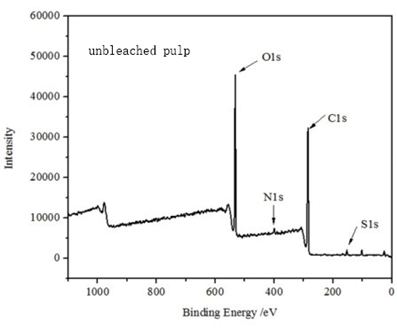(a)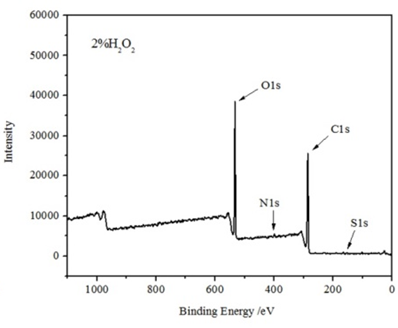(b)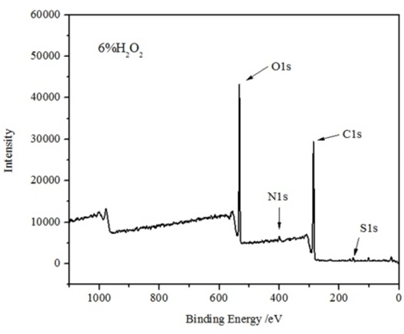(c)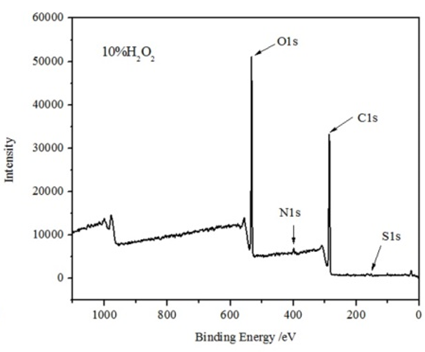(d)

Fig. 8. XPS spectra for mulberry stem SCMP and H2O2 bleached pulp with three treatment levels

The determined atomic composition of unbleached pulp and bleached pulp are shown in Table 6. The change of N and S elements was not obvious before and after bleaching. Within a certain range, increasing the dosage of H2O2, the percentages of N and S elements was increased. However, when the concentration of H2O2 was 10%, the content of N and S elements were decreased.

The decline of S percentage may be caused by partial dissolution of lignin. S/C values reflected lignin sulfonation degree of pulp fiber surface. S/C value of H2O2 bleached pulp was higher than that of unbleached pulp (Table 6), this may be because hemicellulose-related material could be solubilized and removed, thus increasing the relative amount of lignin-type materials at the outer surfaces. This indicates that H2O2 bleaching can improve sulfonation degree of pulp fiber surface.

With the increase of dosage of bleaching agent, the percentage of C was at the surface was reduced, and the percentage of O was increased. Therefore, the O/C ratio also changed. The O/C ratio of the surface of unbleached pulp was 0.42. The O/C ratios were increased to 0.44 (2% H2O2 dosage), 0.46 (6% H2O2 dosage), 0.47 (10% H2O2 dosage) by H2O2 bleaching at increasing levels. The increase of the O/C ratio may result from dissolution of a small amount of extractives or lignin, but the effect of H2O2 bleaching on the content of lignin was unobvious. Therefore, the increase of O/C value was attributed mainly to dissolution of extractives. At the same time, with the increase of H2O2 dosage and the content of oxygen, the amount of carbohydrates was increased, suggested that H2O2 bleaching will reduce the content of extractives and lignin, accompanied by increasing of the relative content of carbohydrates. With the increase of dosage of bleaching agent, the content of extractives and lignin was decreased, the change trend of carbohydrates was opposite.

Table 6. Atomic Composition of Mulberry Stem SCMP and H2O2 Bleached PulpCarbon analysis

Usually the carbons of fibers can be divided into four classes, denoted C1, C2, C3, and C4, indicating different binding modes. A variety of binding mode is shown in Table 7 (Wang et al. 2009). C1, C2, and C3 as the main binding modes.

Table 7. C1s binding modeTable 8. C1s peak area of mulberry stem SCMP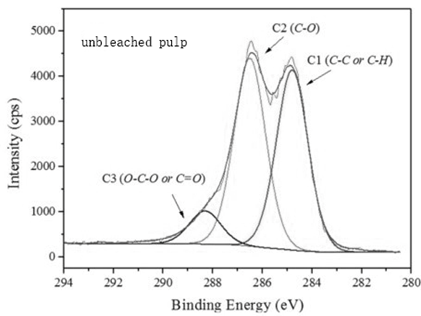(a)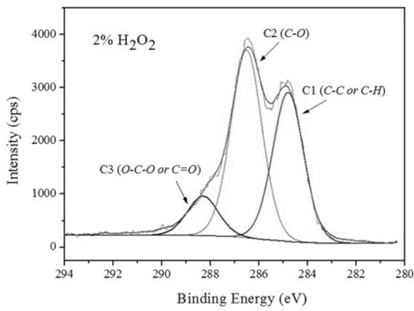(b)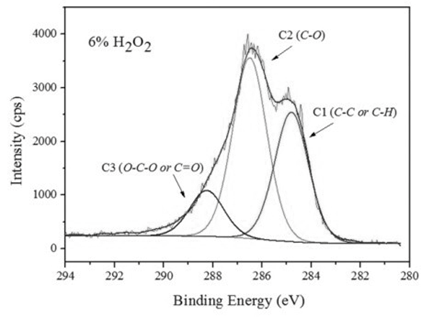(c)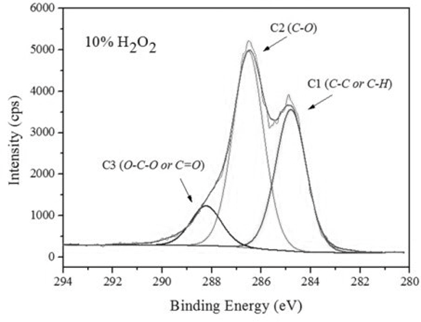(d)

Fig. 9. Carbon characteristic curves of mulberry stem SCMP and H2O2 bleached pulp

Table 8 and Fig. 9 show changes in the carbon groups at the surface of mulberry stem SCMP fibers. The content of C1 was decreased, whereas the contents of C2 and C3 were increased by H2O2 bleaching, suggesting that the content of lignin was reduced and the content of cellulose and hemicellulose were increased. Effects of the H2O2 bleaching were due in part to lignin dissolution and structural changes in the fiber surface.

CONCLUSIONS

1. The Langmuir and Freundlich isotherm models were used to fit the MB adsorption data at different temperatures onto the SCMP of mulberry stems. The R2 values of the Langmuir equation were more than 0.99, whereas the values for the Freundlich equation were between 0.93 and 0.96. The Langmuir adsorption model fit the adsorption data the best. The adsorption process of the SCMP of mulberry stems on MB involved monolayer adsorption. The MB adsorption onto mulberry stem SCMP was negatively impacted as the temperature increased from 20 °C to 40 °C.
2. The adsorption thermodynamics showed that the Gibbs free energy (G), enthalpy (H), and entropy (S) were negative. This indicated that the adsorption process was spontaneous and exothermic. The main driving force was the enthalpy (H).
3. The experimental results were regressed to various adsorption kinetic models: pseudo-first order, pseudo-second order, and intra-particle diffusion. The pseudo-second order model was the best model to explain the MB adsorption kinetics onto mulberry stem SCMP. The adsorption process was controlled by a combination of surface diffusion and intra-particle diffusion.
4. XPS analysis showed that the effect of H2O2 bleaching on the content of surface anion groups was small, at the same time, sulfonation degree of pulp fiber surface was improved, the content of lignin was reduced, the content of cellulose and hemicellulose were increased.

ACKNOWLEDGMENTS

The authors are grateful for the support of the U.S. Department of Biomaterials Research.

REFERENCES CITED

Akhtar, M., Bhanger, M. I., and Iqbal, S. (2006). “Sorption potential of rice husk for the removal of 2,4-dichlorophenol from aqueous solutions: Kinetic and thermodynamic investigations,” J. Hazard. Mater. 128(1), 44-52. DOI: 10.1016/j.jhazmat.2005.07.025

Fardim, P., and Holmbom, B. (2003). “Fast determination of anionic groups in different pulp fibers by MB sorption,” TAPPI J. 2(10), 28-32.

Fardim, P., and Holmbom, B. (2005). “Origin and surface distribution of anionic groups in different papermaking fibers,” Colloid. Surface A 252(2-3), 237-242. DOI: 10.1016/j.colsurfa.2004.10.117

Gao, X. R. (2010). Study on Preparation, Characterization, and Adsorption Properties of Furfural Residue and Modified Furfural Residue, M.S. Thesis, Shenyang University of Technology, Liaoning, China.

He, H. Y. (2015). “Influence and control of chemical mechanical pulp anionic trash,” Paper Science and Technology (6), 24-27.

Han, R., Zhang, J., Zou, W., Shi, J., and Liu, H. (2005). “Equilibrium biosorption isotherm for lead ion on chaff,” J. Hazard. Mater. 125(1-3), 266-271. DOI: 10.1016/j.jhazmat.2005.05.031

Hao, H., Feng, J. G., Ma, C., and Wu, X. (2013). “Adsorption thermodynamics and kinetics of three anionic polymeric dispersants onto imidacloprid particle surface,” CIESC J. 64(10), 3838-3850. DOI: 10.3969/j.issn.0438-1157.2013.10.048

Ho, Y. S., Huang, C. T., and Huang, H. W. (2002). “Equilibrium sorption isotherm for metal ions on tree fern,” Process Biochem. 37(12), 1421-1430. DOI: 10.1016/S0032-9592(02)00036-5

Konn, J., Pranovich, A., and Fardim, P. (2007). “Characterisation and effects of new anionic groups formed during chemithermomechanical pulping of spruce,” Colloids and Surfaces A: Physicochemical and Engineering Aspects. 296(1-3), 1-7.

Kong, L. M., Zhang, T., Wang, P. D., Zhou, X., and Jia, Z. (2015). “Equilibrium and kinetics of phenol adsorption from petrochemical wastewater with activated carbon fiber,” CIESC J. 66(12), 4874-4882.

Kumar, K. V., Ramamurthi, V., and Sivanesan, S. (2005). “Modeling the mechanism involved during the sorption of methylene blue onto fly ash,” J. Colloid Interf. Sci. 284(1), 14-21. DOI: 10.1016/j.jcis.2004.09.063

Li, Z. Q., Qin, Y., Qin, M. H., Liu, N., Xu, Q. H., Fu, Y. J., and Yuan, Z. W. (2012). “Changes of anionic groups in alkaline peroxide peroxide-impregnated aspen chemithermomechanical pulp during subsequent alkaline bleaching,” Carbohydrate Polymers. 88(3), 1041-1046. DOI: 10.1016/j.carbpol.2012.01.063

Li, H. Y., Wang, D. F., and Siaka, D. (2009). “Adsorption thermodynamics and kinetic of tea polyphenols by spherical chitosan resin,” Tea Sci. 29(4), 313-318.

Lorenc-Grabowska, E., and Gryglewicz, G. (2005). “Adsorption of lignite-derived humic acids on coal-based mesoporous activated carbons,” J. Colloid Interf. Sci. 284(2), 416-423. DOI: 10.1016/j.jcis.2004.10.031

Vadivelan, V., and Kumar, K. V. (2005). “Equilibrium, kinetics, mechanism, and process design for the sorption of MB onto rice husk,” J. Colloid Interf. Sci. 286(1), 90-100. DOI: 10.1016/j.jcis.2005.01.007

Von Oepen, B., Kördel, W., and Klein, W. (1989). “Soil preparation for the estimation of adsorption coefficients (Koc) of organic chemicals,” Chemosphere 18(7-8), 1495-1511. DOI: 10.1016/0045-6535(89)90041-6

Von Oepen, B., Kördel, W., and Klein, W. (1991). “Sorption of nonpolar and polar compounds to soils: Processes, measurements, and experience with the applicability of the modified OECD-guideline 106,” Chemosphere 22(3-4), 285-304. DOI: 10.1016/0045-6535(91)90318-8

Wei, X. Z. (2010). Preparation and Adsorption Properties of New Anionic Starch Microspheres, M.S. Thesis, Northwest A&F University, Shanxi, China.

Yang, Q. F., Zhan. H. Y., and Wang. S. F. (2006) “Analysis of bagasse explosion pulp surface XPS,” Papermaking Science and Technology. 25(4), 22-24.

Zhan, H.Y. (2009). Pulping Principle and Engineering, China light industry publisher, Beijing, China.

Zhang, J. J. (2011). Study on Preparation and Properties of Spherical Cellulose Adsorbent, M.S. Thesis, South China University of Technology, Guangdong, China.

#### Zhang, R., Xu, H. D., and Yao, Y. Z. (2012). “Thermodynamic and kinetic studies on the adsorption of cholesterol by Huang Qichao powder,” Food Sci. 33(17), 112-117. DOI: 10.1590/S0104-66322010000200013

Zhang, S. Y. (2009). Study on Mechanism of Solid-Liquid-Gas Interaction in Coal Reservoir, Ph.D. Dissertation, China Mining University, Jiangsu, China.

Article submitted: November 14, 2016; Peer review completed: January 7, 2017; Revised version received: January 26, 2017; Conditional acceptance: January 28, 2017; Accepted: February 2, 2017; Published: February 10, 2017.

DOI: 10.15376/biores.12.2.2452-2465